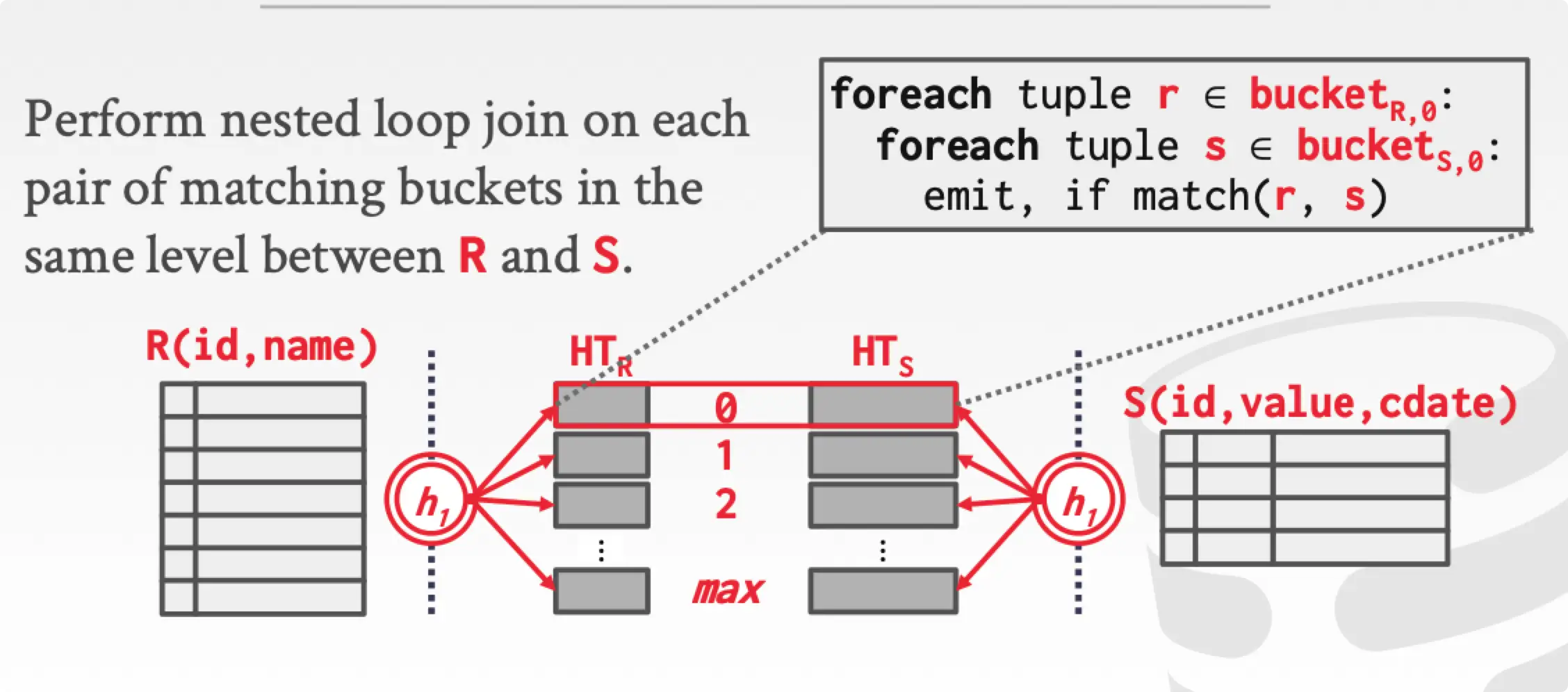• Access：Sequential Scan
• Modifications： Insert, Update, Delete
• Miscellaneous：Nested Loop Join, Hash Join, Aggregation, Limit, Distinct

Access 和 Modifications 没什么好说的，无非是通过 B+ 树进行增删查改工作，这里不说了。

## External Merge Sort

### 阶段一：Sorting

PASS #0：每一次读取 $B$ 个 page 数据，排序，输出文件。总共需要 $\lceil N/B \rceil$ 轮，每一次输出的一个文件称为一个 RUN，故共生成 $\lceil N/B \rceil$个 RUN。

### 阶段二：Merging

PASS #1

PASS #2

PASS #N

$$Number\ Of\ Passes=1+\lceil log_{B-1}\lceil\frac{N}{B}\rceil \rceil$$

$$Total\ I/O\ Cost=2N\cdot (Number\ of\ passes)$$

$2N$ 是因为把一轮都会读取一次全部数据 $N$，一读一写，两次。

### B+ Tree Sort

• Clustered Index：索引和 tuple 存放在一起，通过 B+ 树的性质，直接遍历即可，效果最好。
• Non-Clustered Index：索引和 tuple 分开存放，索引存放着指向 tuple 的指针。虽然索引有序，但是 tuple 不是有序，会涉及大量随机 IO，不采用此方法。

## Aggregation

Aggregation 操作包含 ORDER BYGROUP BYDISTINCT 等。

GROUPY BY 在 Hash Table 中的保存格式是 Group Key->Running Val 。每次插入一个 tuple 时，如果存在对应的 Group Key，则更新 Running Val，根据你的聚合函数处理，比如 sum() 就直接加上去。如果不存在对应的 Group Key，则直接插入 Hash Table。

Running Val 根据你的聚合函数种类存储不同的格式：

## Join

• Early Materialization：在 join 的时候直接把 tuple 连接在一起。好处是后期不用回数据库读取 tuple，缺点是后面的 SELECT 可能只需要几个字段，而你的 tuple 里面可能会包含多余不需要的字段。
• Late Materialization：Join 的时候只拷贝 join keys 对应的 record ID。后期再根据 SELECT 的语句选择性加载 tuple，列式存储的数据库采用这种方法很划算。缺点是后面读取的时候就是随机 IO 了，因为 record ID 指向的数据大概率已经不是连续了。（当然这在按列式存储的数据库里没问题。）

### Join 种类

• Natural Join：将表里面列名相同，且值相同的 tuple 连接在一起，输出的时候不会重复输出相同的列名。SELECT * FROM student NATURAL JOIN takes;
• Join Conditions：Natural Join 是自动连接两个列名相同的 tuple，但是如果我想连接自己指定两个列名，就要使用 ON 语法。SELECT * FROM student JOIN takes ON student.ID = takes.ID;。这种 Join 学术上也叫 equi-join
• Outer Joins：这就是我们传统数据库里面接触的 join。有 left outer join（left join），right outer join（right join） 和 full outer join（full join）。传统 SQL 语法里面一般都直接省略了 outer 关键字。

Inner join … on …：

Left join … on …：

Right join … on …：

Full join … on …：

### Nested Loop Join

Nested loop join 就是用一种很传统的思想，两个 for 循环来实现 join。

$$Cost=M+(m\cdot N)$$

$$Cost=M+N$$

#### Block Nested Loop Join$$Cost=M+(\lceil \frac{M}{B-2} \rceil \cdot N)$$

#### Index Nested Loop Join

$$Cost=M+(m\cdot C)$$

#### 优化

• 选择尽可能小的表作为 outer table。
• 缓存尽可能多的 outer table，针对于 block nested loop join，看 cost 可以看出来。
• 通过遍历 inner table 或 index 的方法来匹配 outer table 的每一个 tuple。

### Sort-Merge Join

1. Sort，inner table 和 outer table 同时根据 join keys 进行排序，可以使用 external merge sort 处理。
2. Merge，弄两个指针，一个 inner table，一个 outer table，同时滑动。
3. 输出的结果已经是按照 join keys 排序的。$$Sort\ Cost(R)=2M\cdot (1+\lceil log_{B-1}\lceil\frac{M}{B}\rceil\rceil)$$

$$Sort\ Cost(S)=2N\cdot (1+\lceil log_{B-1}\lceil\frac{N}{B}\rceil\rceil)$$

$$Merge\ Cost=M+N$$

$$Total\ Cost=Sort+Merge$$

### Hash Join

#### Basic Hash Join

1. Build：扫描 outer table，基于 hash 函数 $h_1(s)$ 建立 hash table。
2. Probe：扫描 inner table，如果 hash table 命中（同样使用 $h_1(s)$ 函数），则输出。

Build 阶段可以同时建立一个 BloomFilter 来帮助查询。BloomFilter 通常很小，可以直接缓存在缓存里面。

#### Grace Hash Join

Basic Hash Join 存在建立 hash table 时 buffer 不足的问题。虽然可以通过换页的方式处理，但是这样的换页是随机的，可能换出的 page 马上被换回来，效率不高。

Grace Hash Join 在 build 阶段，同时建立 outer table 和 inner table 的 hash table，然后将 hash table 划分为多个 partition，每次只比较相同序号的 partition，partition 的比较采用 nested loop join 的方式生成结果。因为相同 join key 的 tuple 必然出现在同一个 partition 里面，所以当两个 partition 比较完后，可以换出 partition，反正不会再需要了。$$Partition\ Phase\ Cost=2(M+N)$$

$$Probing\ Phase=M+N$$

$$Total\ Cost=3(M+N)$$

IO Cost 为一个近似值。

Example 为一个预估时间。

## 发送评论编辑评论

|´・ω・)ノ
ヾ(≧∇≦*)ゝ
(☆ω☆)
（╯‵□′）╯︵┴─┴
￣﹃￣
(/ω＼)
∠( ᐛ 」∠)＿
(๑•̀ㅁ•́ฅ)
→_→
୧(๑•̀⌄•́๑)૭
٩(ˊᗜˋ*)و
(ノ°ο°)ノ
(´இ皿இ｀)
⌇●﹏●⌇
(ฅ´ω`ฅ)
(╯°A°)╯︵○○○
φ(￣∇￣o)
ヾ(´･ ･｀｡)ノ"
( ง ᵒ̌皿ᵒ̌)ง⁼³₌₃
(ó﹏ò｡)
Σ(っ °Д °;)っ
( ,,´･ω･)ﾉ"(´っω･｀｡)
╮(╯▽╰)╭
o(*////▽////*)q
＞﹏＜
( ๑´•ω•) "(ㆆᴗㆆ)

Emoji